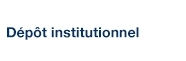# NONLINEAR REDUNDANCY ANALYSIS AND CANONICAL CORRESPONDENCE ANALYSIS BASED ON POLYNOMIAL REGRESSION

Makarenkov, Vladimir et Legendre, Pierre (2002). « NONLINEAR REDUNDANCY ANALYSIS AND CANONICAL CORRESPONDENCE ANALYSIS BASED ON POLYNOMIAL REGRESSION ». Ecology, 83(4), pp. 1146-1161.

Fichier(s) associé(s) à ce document :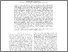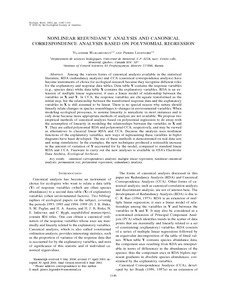Prévisualisation
PDF
Télécharger (305kB)

## Résumé

Among the various forms of canonical analysis available in the statistical literature, RDA (redundancy analysis) and CCA (canonical correspondence analysis) have become instruments of choice for ecological research because they recognize different roles for the explanatory and response data tables. Data table Y contains the response variables (e.g., species data) while data table X contains the explanatory variables. RDA is an extension of multiple linear regression; it uses a linear model of relationship between the variables in X and Y. In CCA, the response variables are chi-square transformed as the initial step, but the relationship between the transformed response data and the explanatory variables in X is still assumed to be linear. There is no special reason why nature should linearly relate changes in species assemblages to changes in environmental variables. When modeling ecological processes, to assume linearity is unrealistic in most instances and is only done because more appropriate methods of analysis are not available. We propose two empirical methods of canonical analysis based on polynomial regression to do away with the assumption of linearity in modeling the relationships between the variables in X and Y. They are called polynomial RDA and polynomial CCA, respectively, and may be viewed as alternatives to classical linear RDA and CCA. Because the analysis uses nonlinear functions of the explanatory variables, new ways of representing these variables in biplot diagrams have been developed. The use of these methods is demonstrated on real data sets and using simulations. In the examples, the new techniques produced a noticeable increase in the amount of variation of Y accounted for by the model, compared to standard linear RDA and CCA. Freeware to carry out the new analyses is available in ESA’s Electronic Data Archive, Ecological Archives.

Type: Article de revue scientifique canonical correspondence analysis; multiple linear regression; nonlinear canonical analysis; permutation test; polynomial regression; redundancy analysis. Faculté des sciences > Département d'informatique Vladimir Makarenkov 16 févr. 2016 15:03 20 avr. 2016 19:36 http://archipel.uqam.ca/id/eprint/7833

## Statistiques

Voir les statistiques sur cinq ans...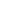RECHERCHER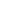PARCOURIR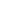LIBRE ACCÈS